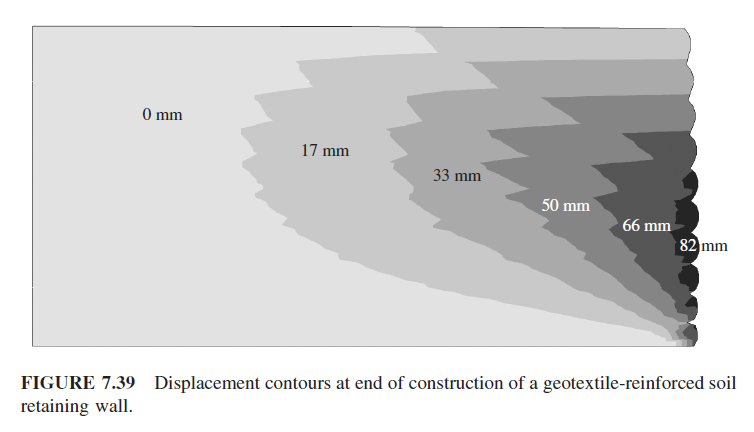آخرین دیدگاه‌ها
پکیج آموزش پیشرفته آباکوس
پکیج جامع آباکوس ویژه رشته مکانیک
پکیج جامع آباکوس ژئوتکنیک و عمران

Sequential Construction of a Geotextile-Reinforced Soil Retaining Wall Abaqus

This example been chosen from Example 7.5 Sam Helwany book

geosynthetic-reinforced soil (GRS) walls are used when a sudden change of elevation is desired Geosynthetics are made of polymers such as polyester, polyethylene

Two types of geosynthetics are widely used for soil reinforcement 1-geotextiles 2-geogrids

The part of the backfill that contains the reinforcement is called the reinforced soil, and the part of the backfill behind that is called the retained soil , see the picture below

In this example, we simulate sequential construction (layer by layer) of a geotextile-reinforced soil retaining wall in Abaqus software

The wall is constructed in 10 equal layers each 0.3 m thick. Each layer is constructed in a 1-second period, during which the self-weight of the soil layer is applied. The total construction time is 10 seconds

The geotextile reinforcement is 3 mm thick and has a elastic modulus of 20,000 kPa

For simulation Geotextile ,we use Beam elements

The soil is assumed to be elastoplastic, obeying the cap model with cap hardening properties

In the first calculation step, the entire wall is removed from the finite element mesh except for the bottom soil layer, the first reinforcement layer, and the first skin layer. Then the layers are added, one by one, in subsequent calculation steps. The self-weight of each layer is applied using the gravity optionHorizontal displacement contours at end of construction of a geotextile-reinforced soil retaining wall by Sam Helwany book